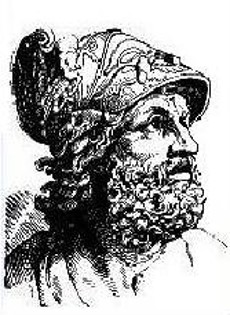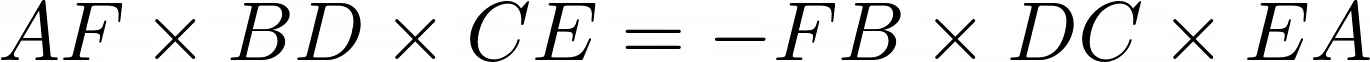## MenelausMenelaus of Alexandria was a Greek mathematician and astronomer, the first to recognize geodesics on a curved surface as natural analogs of straight lines.

Menelaus’ TheoremMenelaus’s theorem is a proposition about triangles in plane geometry. Given a triangle ABC, and a transversal line that crosses BC, AC, and AB at points D, E, and F respectively, with D, E, and F distinct from A, B, and C, then